﻿ 辽宁葫芦岛市新旧暴雨强度公式对比及暴雨雨型分析
 期刊检索： 题目 作者 关键词
 暴雨灾害2017, Vol. 36Issue (3): 251-258.  DOI: 10.3969/j.issn.1004-9045.2017.03.008### DOI

10.3969/j.issn.1004-9045.2017.03.008

### 文章历史

1. 沈阳区域气候中心，沈阳 110166;
2. 辽宁广播电视大学，沈阳 110034

Comparison of new and old rainstorm intensity formulas and the designed rainstorm pattern for Huludao
, GONG Qiang1, LI Yang2, GU Zhengqiang1, LIN Na1, CHAO Hua1, XU Hong1, LI Qian1
1. Shenyang Regional Climate Center, Shenyang 110016;
2. Liaoning Radio and TV University, Shenyang 110034
Abstract: Based on the minute precipitation data from 1973 to 2014 at Huludao, we establish rainstorm statistical samples. The revision of rainstorm intensity formula at Huludao was carried out by the annual maximum value method. The probability curves were followed by P-Ⅲ distribution, and the formula parameters were calculated. The rain intensity calculated by the old formula from 5 to 30 minutes of each return period are lager than that calculated by the new method. The rain intensity calculated by the new and old formula of 45 minutes of each return period are basically equivalent. The rain intensity calculated by the new formula from 60 to 180 minutes of each return period are lager than that by the old method. Based on these results, the designed rainstorm pattern at Huludao is studied. Using the coefficient of rain peak position of 0.32, and the designed rainstorm pattern of the Chicago, the accumulated precipitation of rainstorm pattern of return period of 2 a lasted 60, 90, 120, 150, 180 minutes are between 38.73 to 66.99 mm. It increases slowly early in the precipitation, but increases faster around the rain peak, then increases slowly again.
Key words: rainstorm    rainstorm intensity formula    rainstorm pattern    rain peak position    Huludao

1 资料与方法 1.1 资料

1.2 方法 1.2.1 暴雨强度公式计算

(1) 暴雨选样。年最大值法选样选取降雨资料样本中各降雨历时雨量的逐年最大值，即42个样本。

(2) 频率分布曲线拟合。文献推荐皮尔逊Ⅲ型(简称P-Ⅲ型)分布曲线、耿贝尔分布曲线和指数分布曲线，本文从中选取拟合效果较好、误差最小的概率分布函数曲线，推求暴雨强度公式。上述三种理论概论曲线的数学表达式以及原理等可参见文献。

(3) 暴雨强度公式及参数求解。暴雨强度公式定义为

 $q = \frac{{167{A_1}\left( {1 + C\lg P} \right)}}{{{{\left( {t + b} \right)}^n}}}$ (1)

 $\ln q = \ln 167{A_1} + \ln \left( {1 + C\lg P} \right) - n\ln \left( {t + b} \right)$ (2)

y = ln q - ln(1 + ClgP)，b0 = ln 167A1b1 = -nx = ln(t + b)，即得：

 $y = {b_0} + {b_1}x$ (3)

 $\bar \sigma = \frac{1}{{{m_0}}}\sum\limits_{j = 1}^{{m_0}} {\sqrt {\frac{1}{m}\sum\limits_{i = 1}^m {{{\left( {{q_{ij}} - {{q'}_{ij}}} \right)}^2}} } }$ (4)

 ${X_m} = \sqrt {\frac{1}{n}\sum\limits_{i = 1}^n {{{\left( {\frac{{{{R'}_i} - {R_i}}}{{{t_i}}}} \right)}^2}} }$ (5)

 ${U_m} = \sqrt {\frac{1}{n}\sum\limits_{i = 1}^n {{{\left( {\frac{{{{R'}_i} - {R_i}}}{{{R_i}}}} \right)}^2}} } \times 100\%$ (6)

1.2.2 芝加哥雨型设计方法

(1) 雨峰位置系数确定。芝加哥雨型以暴雨强度公式为基础设计典型降雨过程。将各降雨历时的逐年降雨过程样本，以5 min为间隔进行分段，统计降雨过程的雨峰位置系数。

 $r={}^{t}\!\!\diagup\!\!{}_{T}\;$ (7)

(2) 芝加哥降雨模型。雨峰前、后瞬时降雨强度可由以下两个公式计算：

 $i\left( {{t_{{b}}}} \right) = \frac{{A\left[ {\frac{{\left( {1 - n} \right){t_b}}}{r} + b} \right]}}{{{{\left[ {\left( {\frac{{{t_{{b}}}}}{r}} \right) + b} \right]}^{n + 1}}}}$ (8)
 $i\left( {{t_a}} \right) = \frac{{A\left[ {\frac{{\left( {1 - n} \right){t_a}}}{r} + b} \right]}}{{{{\left[ {\left( {\frac{{{t_{{a}}}}}{{1 - r}}} \right) + b} \right]}^{n + 1}}}}$ (9)

2 新暴雨强度公式编制 2.1 估算方法的优选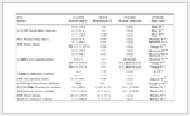表 1 多种暴雨强度公式推算结果精度检验 Table 1 The accuracy test of storm intensity formula derived by different methods
2.2 暴雨强度公式

 $q = \frac{{756.649 \times \left( {1 + 0.984\lg P} \right)}}{{{{\left( {t + 5.483} \right)}^{0.528}}}}$ (10)

 $q = \frac{{658.773 \times \left( {1 + 0.737\lg P} \right)}}{{{{\left( {t + 1.950} \right)}^{0.469}}}}$ (11)

 $q = \frac{{1\;878 \times \left( {1 + 0.8\lg P} \right)}}{{{{\left( {t + 6} \right)}^{0.732}}}}$ (12)

3 新旧暴雨强度公式差异及原因分析 3.1 暴雨强度公式对比

 ${\varepsilon _1} = \frac{{{i_m} - {i_o}}}{{{i_o}}} \times 100\%$ (13)

 ${\varepsilon _2} = \frac{{{i_n} - {i_o}}}{{{i_o}}} \times 100\%$ (14)

 ${\varepsilon _3} = \frac{{{i_m} - {i_n}}}{{{i_m}}} \times 100\%$ (15)表 2 年最大值法、年多个样法新编暴雨强度公式和旧暴雨强度公式的雨强差值比率 Table 2 Difference ratios of rainstorm intensity calculated by the new formula of maximum method, annual multisampling method and the old formula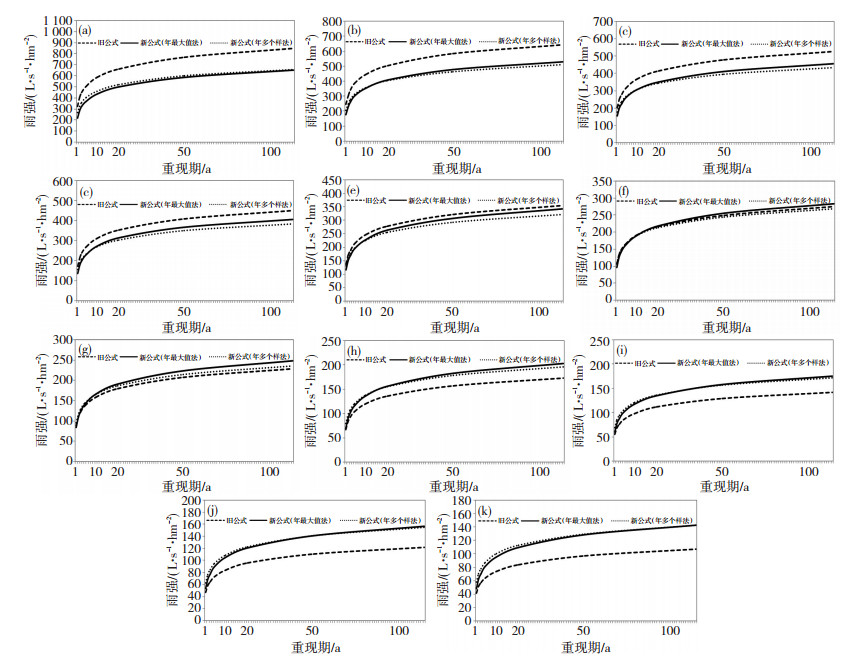图 1 年最大值法、年多个样法新编暴雨强度公式和旧暴雨强度公式各降雨历时雨强对比 (a) 5 min, (b) 10 min, (c) 15 min, (d) 20 min, (e) 30 min, (f) 45 min, (g) 60 min, (h) 90 min, (i) 120 min, (j) 150 min, (k) 180 min Fig. 1 Comparison of rainstorm intensity of different rainfall durations calculated by the new formula of maximum method, annual multisampling method and the old formula (a) 5 min, (b) 10 min, (c) 15 min, (d) 20 min, (e) 30 min, (f) 45 min, (g) 60 min, (h) 90 min, (i) 120 min, (j) 150 min, (k) 180 min

3.2 原因分析

3.2.1 年日最大降雨量变化特征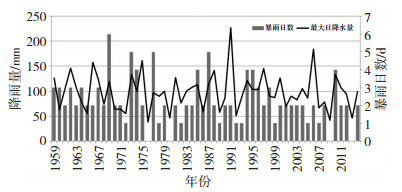图 2 葫芦岛1959—2014年的年最大日降雨量及暴雨日数 Fig. 2 The annual maximum daily precipitation amount and rainstorm days at Huludao from 1959 to 2014
3.2.2 各历时最大降雨年变化特征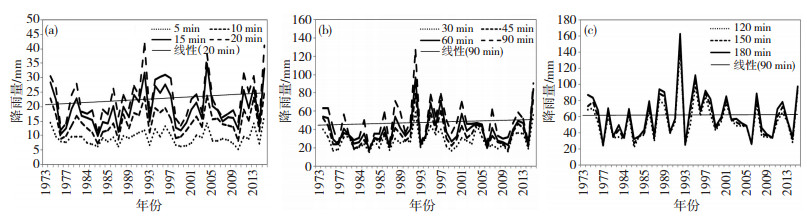图 3 葫芦岛1973—2014年各历时最大降雨量 Fig. 3 The maximum precipitation of different rainfall durations at Huludao from 1973 to 2014表 3 葫芦岛1973—2014年各历时最大降雨量的趋势系数和最大降雨量变率（最大降雨量变化占最大降雨量平均值的比率）的气候倾向率(单位：%·(10a-1)) Table 3 The trend coefficient of maximum precipitation and climate tendency rate (unit: %·(10a-1)) of variety rate of maximum precipitation of different rainfall durations at Huludao from 1973 to 2014

4 短历时暴雨雨型

4.1 暴雨雨峰位置系数表 4 不同降雨历时的时长参考区间、雨量阀值指标及对应的雨峰位置系数 Table 4 The reference continuous interval, threshold index of rainfall and rain peak position coefficient of different rainfall durations
4.2 短历时暴雨雨型推求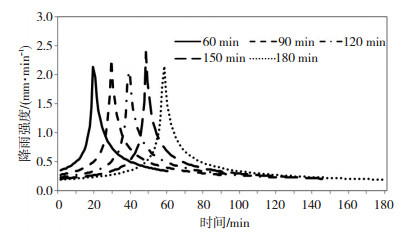图 4 芝加哥法推求重现期2 a的各降雨历时暴雨雨型分布 Fig. 4 The distribution of designed rainstorm patterns of Chicago method of different rainfall durations of 2 a return period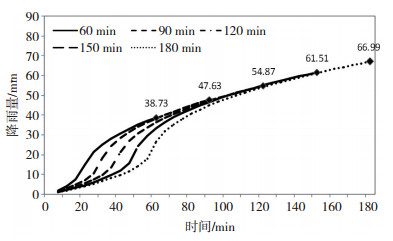图 5 芝加哥法推求重现期2 a的各降雨历时累计降雨量 Fig. 5 Accumulated precipitation of Chicago method of different rainfall durations of 2 a return period
5 适用性分析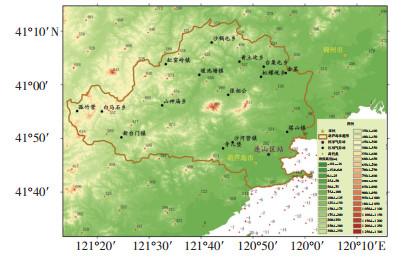图 6 葫芦岛市地形特征及气象站位置 Fig. 6 Terrain feature and location of meteorological stations at Huludao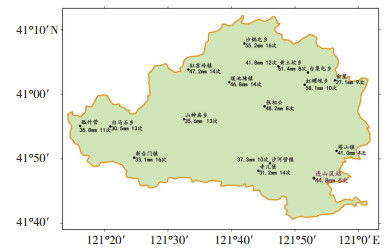图 7 葫芦岛市小时最大降雨量(单位: mm)和小时暴雨次数分布特征 Fig. 7 Distribution characteristics of maximum hourly precipitation (unit: mm) and the number of hourly rainstorm at Huludao

6 结论和讨论

(1) 根据年最大值法和年多个样法，采用P-Ⅲ型分布曲线拟合得到的暴雨强度公式精度均能满足导则要求，推求的暴雨强度公式计算的雨强差异较小。根据估算方法优选结果，采用年最大值法P-Ⅲ型分布曲线拟合的新暴雨强度公式精度最高，因此推荐葫芦岛市暴雨强度公式选用式（10）。

(2) 新暴雨强度公式5~30 min历时各重现期雨强较旧暴雨强度公式偏小，45 min历时各公式各重现期的雨强基本相当，60~180 min历时各重现期雨强较旧暴雨强度公式偏大。

(3) 近年来，葫芦岛市暴雨出现概率虽有所减小，但暴雨强度有增强趋势，各历时最大降雨量均有一定的增大趋势。暴雨变化特征显著，导致新、旧暴雨强度公式的计算结果差异较大，新暴雨强度公式适用于当前暴雨特征。

(4) 基于芝加哥雨型法得到相应的短历时暴雨雨型，葫芦岛市短历时暴雨雨峰位置系数为0.32，以重现期2 a为例，历时60、90、120、150、180 min的峰值降雨强度在2.048~2.391 mm·min-1，雨峰出现时间分别为第19、29、39、48、58 min，累计降雨量在38.73~66.99 mm，各历时降雨均在初期累计降雨增长较慢，峰前后增长速度较快，之后增速明显放缓。

  丁一汇, 任国玉, 石广玉, 等. 气候变化国家评估报告(1):中国气候变化的历史和未来趋势[J]. 气候变化研究进展, 2006, 2(1): 3-8. DOI:10.3969/j.issn.1673-1719.2006.01.001  翟盘茂, 李蕾. IPCC第五次评估报告反映的大气和地表观测变化[J]. 气候变化研究进展, 2014, 10(1): 20-24. DOI:10.3969/j.issn.1673-1719.2014.01.004  谢五三, 王胜. 近40 a淮河流域暴雨特征分析[J]. 暴雨灾害, 2010, 12(4): 377-380. DOI:10.3969/j.issn.1004-9045.2010.04.013  吴滨, 文明章, 李玲, 等. 福建省不同短历时暴雨时空分布特征[J]. 暴雨灾害, 2015, 6(2): 153-159. DOI:10.3969/j.issn.1004-9045.2015.02.007  岑国平, 沈晋, 范荣生. 城市设计暴雨雨型研究[J]. 水科学进展, 1998, 9(1): 41-46. DOI:10.3321/j.issn:1001-6791.1998.01.007  王敏, 谭向诚. 北京城市暴雨和雨型的研究[J]. 水文, 1994(3): 1-6.  张秉祥, 陈静, 韩军彩, 等. 石家庄市城区暴雨强度公式修正方法对比分析[J]. 干旱气象, 2014, 32(4): 671-676.  陈正洪, 王海军, 张小丽. 深圳市新一代暴雨强度公式的研制[J]. 自然灾害学报, 2007, 16(3): 29-34. DOI:10.3969/j.issn.1004-4574.2007.03.006  庄智福, 王珂清, 杨杰, 等. 镇江市新一代暴雨强度公式编制及雨型设计[J]. 气象科学, 2015, 35(4): 506-513.  成丹, 陈正洪, 方怡. 宜昌市区短历时暴雨雨型特征[J]. 暴雨灾害, 2015, 34(3): 249-253. DOI:10.3969/j.issn.1004-9045.2015.03.007  住房和城乡建设部, 中国气象局. 城市暴雨强度公式编制和设计暴雨雨型确定技术导则[M]. 北京: 气象出版社, 2014: 1-25.  Amell V, Harremoes P, Jensen M, et al. Review of rainfall data application for design and analysis[J]. Water Sci. Technol, 1984, 16(8-9): 1-45. DOI:10.2166/wst.1984.0176  顾俊强, 徐集云, 陈海燕, 等. 暴雨强度公式参数估计及其应用[J]. 南京气象学院学报, 2000, 23(1): 63-67. DOI:10.3969/j.issn.1674-7097.2000.01.010  陈正洪, 王海军, 张小丽, 等. 水文学中雨强公式参数求解的一种最有方法[J]. 应用气象学报, 2007, 18(2): 237-241. DOI:10.3969/j.issn.1001-7313.2007.02.015  金家明. 城市暴雨强度公式编制及其应用方法[J]. 中国市政工程, 2010(1): 38-39, 41. DOI:10.3969/j.issn.1004-4655.2010.01.015  植石群, 宋丽莉, 罗金铃, 等. 暴雨强度计算系统及其应用[J]. 气象, 2000, 26(6): 30-33. DOI:10.3969/j.issn.1000-0526.2000.06.007  中华人民共和国住房和城乡建设部. 室外排水设计规范(GB50014-2006, 2014年版)[M]. 北京: 中国计划出版社, 2014.